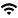# Industrial & Enterprise Systems Engineering Calendar

Back to Listing

## Final Exam for Qi Zhao

Event Type
Meeting
Sponsor
ISE Graduate Programs
Location
please contact Lauren Redman for event link
VirtualDate
Jun 21, 2021   3:30 - 5:30 pm
Contact
Lauren Redman
E-Mail
ise-grad@illinois.edu
Views
36
Originating Calendar
ISE Academic Programs

Committee:

Chronopoulou, Alexandra (director of research)

Feng, Liming (chair)

Sirignano, Justin A (member)

Sreenivas, Ramavarapu S (member)

Financial Engineering

Fractional Stochastic Volatility Models: Approximation, Calibration and Hedging

The area of modeling stochastic volatility using continuous time models has a long history and is always an interesting and vibrant area in financial mathematics, where the dynamics of the stock is a diffusion driven by Brownian motion and the dynamic of the volatility is associated with a diffusion driven also by another Brownian motion, instead of a fixed constant as in Black-Scholes model. However recent works have pointed out that there are some observations that the semimartinagale or Markovian models cannot explain, for example volatility persistence or the roughness of the sample paths of volatilities. And that is when the fractional stochastic volatility models are introduced. Our work about fractional stochastic volatility models mainly covers three different directions: hedging, calibration and approximation.

First in our work, we propose a delta-hedging strategy for a long memory stochastic volatility model (LMSV). This is a model in which the volatility is driven by a fractional Ornstein-Uhlenbeck process with Hurst Index H greater than 1/2. We need to notice that perfect hedging cannot be achieved in the stochastic volatility framework and it is the same with the non-Markovian fractional volatility framework, and thus we can only study imperfect delta-hedging strategy. We first proved the existence of this strategy by establishing the differentiability of the option price with respect to the underlying asset price. We also compute the so-called hedging bias, i.e. the difference between the Black-Scholes Delta and the LMSV Delta, and we determine when a European-type option is over-hedged or under-hedged.

Second in our work, to address the concern whether roughness can only be observed in the high frequency data, we first propose a new volatility proxy framework using low frequency cumulative option trading entries. Upon that framework we estimate the Hurst Index of the volatility and verify that the volatility exhibits very rough behavior even in the low frequency (daily) settings, which corroborates that roughness should always be taken into consideration when modeling the volatility for option pricing and calibration. In the case of pricing option in the long run we observe that the hurst number varies largely, suggesting the hurst number is a local parameter and is not appropriate to be taken as a constant in the long setting.

Third in our work, we consider the volatility model driven by fractional Ornstein-Unlenbeck process, with Hurst Index H less than 1/2. We propose a point process approximation scheme to both the volatility process and the asset price process, and we first prove a Donsker type theorem for the convergence to fractional Ornstein-Uhlenbeck process, and with that theorem we prove the weak convergence of the scheme to the log-price process. We also take into account the correlation between the volatility and the stock process, which is also termed as leverage effect. This work will be conducted with respect to Skorohod topology and we will not have interpolating term. The result can be generalized to all point process with jumps consisting of bounded random variables.

link for robots only